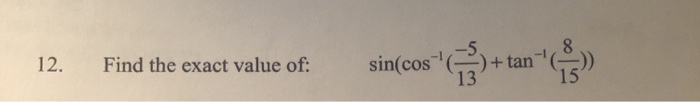# Let sin alpha = 5/13 where 90° is less than or equal to alpha is less than or equal to 180° and cos beta = -24/25 where 180° is less than or equal to beta is less than or equal to 270° Find cos (alpha - beta) and tan (alpha + beta)

Let sin alpha = 5/13 where 90° is less than or equal to alpha is less than or equal to 180° and cos beta = -24/25 where 180° is less than or equal to beta is less than or equal to 270°
Find cos (alpha - beta) and tan (alpha + beta)

This Homework Help Question: "Let sin alpha = 5/13 where 90° is less than or equal to alpha is less than or equal to 180° and cos beta = -24/25 where 180° is less than or equal to beta is less than or equal to 270° Find cos (alpha - beta) and tan (alpha + beta)" No answers yet.

We need 10 more requests to produce the answer to this homework help question. Share with your friends to get the answer faster!

0 /10 have requested the answer to this homework help question.

Once 10 people have made a request, the answer to this question will be available in 1-2 days.
All students who have requested the answer will be notified once they are available.
##### Add Answer of: Let sin alpha = 5/13 where 90° is less than or equal to alpha is less than or equal to 180° and cos beta = -24/25 where 180° is less than or equal to beta is less than or equal to 270° Find cos (alpha - beta) and tan (alpha + beta)
Similar Homework Help Questions
• ### For which angles beta, with 0 degrees less than/equal to beta less than/equal to 180 degrees, is sin 3beta equal to -1

For which angles beta, with 0 degrees less than/equal to beta less than/equal to 180 degrees,is sin 3beta equal to -1?a) 30 degress and 150 degreesb) 60 and 120 degreesc) 90 degreesd) 0 and 120 degreesI think it is C because sin of (3 x 90 = 270) 270 degrees is -1?Did I do this right? Or did I do something that cannot be done?Thank you.

• ### Find exact value under following conditions: sin alpha =5/6, pi/2 < alpha < pi, and tan beta = 3/7, pi < beta < 3 pi/2 Find cos (alpha + beta), sin (alpha + beta), and tan (alpha + beta)

Find exact value under following conditions: sin alpha =5/6, pi/2 < alpha < pi, and tan beta = 3/7, pi < beta < 3 pi/2 Find cos (alpha + beta), sin (alpha + beta), and tan (alpha + beta)

• ### Given that sin alpha=4/sqrt65, pi/2<alpha<pi, and cos beta=-3/sqrt13, tan beta>0, find the exact value of tan(alpha+beta)

Given that sin alpha=4/sqrt65, pi/2<alpha<pi, and cos beta=-3/sqrt13, tan beta>0, find the exact value of tan(alpha+beta)

• ### Solve each equation for o is less than and/or equal to theta is less than and/or equal to 360 -- sin^2x = 1 = cos^2x -- Work: cos^2x - cos^2x = 0 0 = 0 -- Textbook Answers: 90 and 270 -- Btw, how would you isolate for cos^2x = 0

Solve each equation for o is less than and/or equal to theta is less than and/or equal to 360--sin^2x = 1 = cos^2x--Work:cos^2x - cos^2x = 00 = 0--Textbook Answers:90 and 270--Btw, how would you isolate for cos^2x = 0? Would it be...x = cos^-1 square root of _____ ?

• ### Given: csc = - 17/15 where 270 < 360 cot = -3/4 where 90 < 180 Find the exact value of sin

Given:csc = - 17/15 where 270 < 360 cot = -3/4 where 90 < 180Find the exact value of sin. Show all work.

• ### Find the exact value of: sin(cos^-1(-5/13) + tan^-1(8/15 12. Find the exact value of: sin(cos +...Find the exact value of: sin(cos^-1(-5/13) + tan^-1(8/15 12. Find the exact value of: sin(cos + tan 13

• ### 9(tan(2a)+2cos(a)=0 interval 0 less than or equal to a less than or equal to 2pi

9(tan(2a)+2cos(a)=0 interval 0 less than or equal to a less than or equal to 2piSolve each equation...trig equations for linear sin and cos

• ### how i solve this two equations ib order to find alpha and beta 2=cos(alpha)+1.341cos(beta) 2=sin(alpha)-1.341sin(beta)

how i solve this two equations ib order to find alpha and beta2=cos(alpha)+1.341cos(beta)2=sin(alpha)-1.341sin(beta)

• ### cos alpha = - square root of 3 / 5 and alpha is in the third quadrant. Find exact values for sin...

cos alpha = - square root of 3 / 5 and alpha is in the third quadrant. Find exact values for sin alpha and tan alpha.

• ### 1)Find cos theta if sin theta=3/5 and 90 degrees<theta<180 degrees

1)Find cos theta if sin theta=3/5 and 90 degrees<theta<180 degrees.A)4/5B)-4/5C)square root of 34/5D)-square root of 34/5I chose A2)Simplify:1-csc^2theta/cot^2thetaA)-1B)1C)tan^2thetaD)1/sin^4thetaI chose C3)Simplify:-5(cot^2theta-csc^2theta)A)5B)-5C)-5csc^2 thetaD)5csc^2 thetaI chose B4)Which expression is not equivalent to 1?A)sin^2theta+cot^2thetasin^2thetaB)sin^2theta/1-costheta -1C)sec^2theta+tan^2thetaD)cot^2thetasin^2theta/cos^2thetaI don't know5)Which expression is equivalent to tan theta-sec theta/sin theta?A)-cot thetaB)cot thetaC)tan theta-cot thetaD)tan theta-sec^2thetaI don't know

Free Homework App